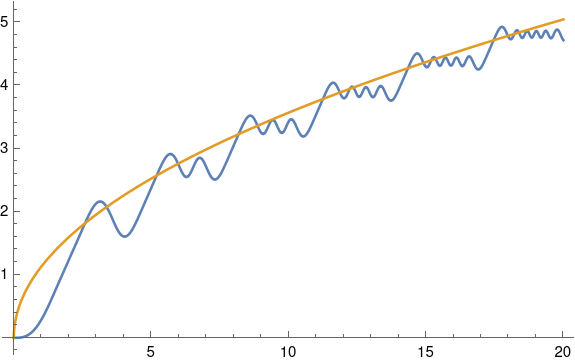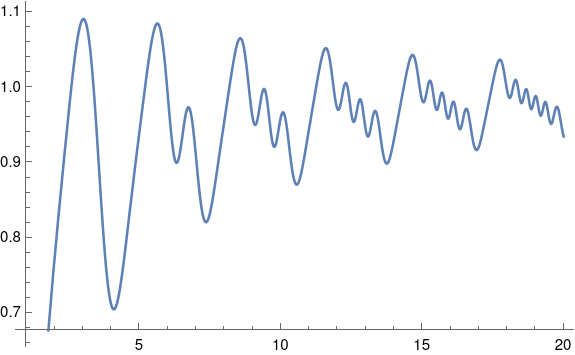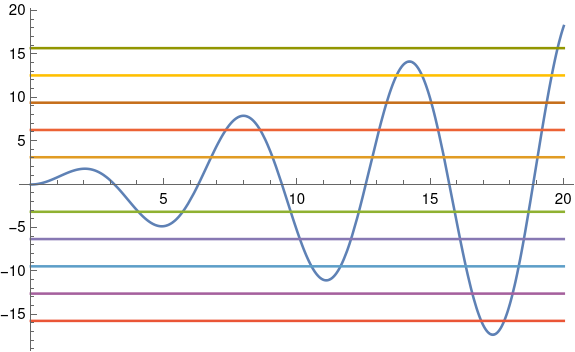# Glasser’s function

I recently ran across an article on MathWorld about Glasser’s function. The function is defined byHere’s a plot of G(x) along with its asymptotic value 2√(x/π).This plot was made with the following Mathematica commands.

    G[x_] := NIntegrate[Sin[t Sin[t]], {t, 0, x}]
Plot[{G[x], 2 Sqrt[x/Pi]}, {x, 0, 20}]


According to MathWorld,

The integral cannot be done in closed form, but has a number of remarkable properties, the foremost of which is that the first “hump” has a single subhump, the second hump has two subhumps, and so on.

To get a clearer view of these humps and “subhumps”, let’s subtract off the asymptotic value.Plot[{G[x]/( 2 Sqrt[x/Pi])}, {x, 1, 20}, PlotPoints -> 100]

Ordinarily Mathematica chooses the number of plot points well, but the default led to an artificial flat spot on the graph so I manually increased the number of points.

What MathWorld is calling a “hump” is a record maximum as you view the plot from left to right. That is, each one is a global maximum over the interval [0, x + ε] where x is the location of the hump.

The humps seem to be evenly spaced. Is that the case?

By the fundamental theorem of calculus, the derivative of G(x) is the integrand of the integral defining G.and G‘ is zero when x sin(x) is an integer multiple of π. To get an idea where the local extrema of G(x) are, we’ll plot x sin(x) and some integer multiples of π.This was produced with the following code.

    Plot[{x Sin[x], Pi, -Pi, 2 Pi, -2 Pi, 3 Pi, -3 Pi,
4 Pi, -4 Pi, 5 Pi, -5 Pi}, {x, 0, 20}]


Note that between the first two zeros of sin(x), i.e. between x = 0 and x = π, there are no points where x sin(x) is an integer multiple of π. Between the next two zeros of sin(x), between π and 2π, the function x sin(x) takes on an integer multiple of π twice. The blue line plotting x sin(x) crosses the green line below the x axis twice. The function takes on integer multiples of π 4 times between x = 2π and 3π, 6 times between x = 3π and 4π etc.

Apparently between n π and (n + 1) π the function x sin(x) takes on integer multiples of π 2n times, which means G'(x) is zero 2n times, which means G has 2n local extrema.

If we number the locations where x sin(x) is an integer multiple of π, starting from 0, then x = 0 is the 0th solution, x = π is the 1st solution, x = 2π is the 4th solution, etc. In general, x = nπ is the location of solution number n².

The first “hump” is at solution 1 to x sin(x). The second hump is at solution 3. The third is at solution 7. The fourth is at solution 13 etc. Each time there is one more “subhump” between humps, two more extrema, between humps than before.

The nth hump is located at the (n² – n + 1)st place where x sin(x) is an integer multiple of π.

The locations of the humps between x = 0 and x = 20 are as follows:

    3.14159
5.69936
8.60636
11.62042
14.68050
17.76473


The first differences of these values are

    2.55778
2.90700
3.01406
3.06008
3.08423


and so the distance between humps is roughly 3 and is increasing slowly. Perhaps it continues to increase, asymptotically approaching a constant distance.